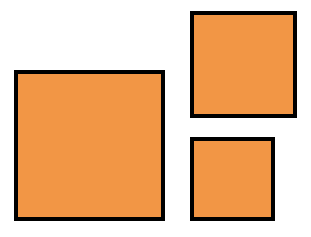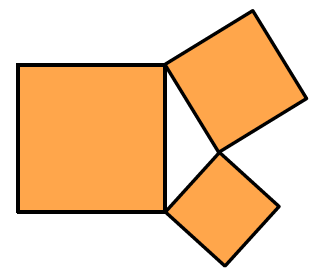### Home > CC3MN > Chapter 9 > Lesson 9.1.1 > Problem9-7

9-7.

Jenna is working with three squares. Their areas are $16 \text{ cm}^{2}, 9 \text{ cm}^{2}$, and $36 \text{ cm}^{2}$. She thinks they will make an obtuse triangle. Do you agree? Explain your reasoning.

What are the values of the sides of each square?

Try to predict what kind of triangle can be made from the squares below.The sides of the squares are $4$ cm, $3$ cm, and $6$ cm.
These values make up the values of the sides of the triangle.

What kind of triangle do these squares make?